HomeLearnHow-toPreparing Time Series data for Analysis Tools with \$densify and \$fill

# Preparing Time Series data for Analysis Tools with \$densify and \$fill

Updated: Apr 06, 2022 |

Published: Apr 06, 2022

• MongoDB
• Atlas
• Time Series
• ...

By John Page

Time series data refers to recordings of continuous values at specific points in time. This data is then examined, not as individual data points, but as how a value either changes over time or correlates with other values in the same time period.

Normally, data points would have a timestamp, one or more metadata values to identify what the source of the data is and one or more values known as measurements. For example, a stock ticker would have a time, stock symbol (metadata), and price (measurement), whereas aircraft tracking data might have time, tail number, and multiple measurement values such as speed, heading, altitude, and rate of climb. When we record this data in MongoDB, we may also include additional category metadata to assist in the analysis. For example, in the case of flight tracking, we may store the tail number as a unique identifier but also the aircraft type and owner as additional metadata, allowing us to analyze data based on broader categories.

Analysis of time series data is usually either to identify a previously unknown correlation between data points or to try and predict patterns and thus, future readings. There are many tools and techniques, including machine learning and Fourier analysis, applied to examine the changes in the stream of data and predict what future readings might be. In the world of high finance, entire industries and careers have been built around trying to say what a stock price will do next.

Some of these analytic techniques require the data to be in a specific form, having no missing readings and regularly spaced time periods, or both, but data is not always available in that form.

Some data is regularly spaced; an industrial process may record sensor readings at precise intervals. There are, however, reasons for data to be missing. This could be software failure or for external social reasons. Imagine we are examining the number of customers at stations on the London underground. We could have a daily count that we use to predict usage in the future, but a strike or other external event may cause that traffic to drop to zero on given days. From an analytical perspective, we want to replace that missing count or count of zero with a more typical value.

Some data sets are inherently irregular. When measuring things in the real world, it may not be possible to take readings on a regular cadence because they are discrete events that happen irregularly, like recording tornados or detected neutrinos. Other real-world data may be a continuous event but we are only able to observe it at random times. Imagine we are tracking a pod of whales across the ocean. They are somewhere at all times but we can only record them when we see them, or when a tracking device is within range of a receiver.

Having given these examples, it’s easier to explain the actual functionality available for densification and gap-filling using a generic concept of time and readings rather than specific examples, so we will do that below. These aggregation stages work on both time series and regular collections.

## #Add missing data points with \$densify

The aggregation stage `\$densify` added in MongoDB 5.2 allows you to create missing documents in the series either by filling in a document where one is not present in a regularly spaced set or by inserting documents at regularly spaced intervals between the existing data points in an irregularly spaced set.

Imagine we have a data set where we get a reading once a minute, but sometimes, we are missing readings. We can create data like this in the mongosh shell spanning the previous 20 minutes using the following script. This starts with creating a record with the current time and then subtracts 60000 milliseconds from it until we have 20 documents. It also fails to insert any document where the iterator divides evenly by 7 to create missing records.

``1db=db.getSiblingDB('tsdemo')2db.data.drop()34let timestamp =new  Date()5for(let reading=0;reading<20;reading++) {6   timestamp = new Date(timestamp.getTime() - 60000)7   if(reading%7) db.data.insertOne({reading,timestamp})8}``

Whilst we can view these as text using db.data.find() , it’s better if we can visualize them. Ideally, we would use MongoDB Charts for this. However, these functions are not yet all available to us in Atlas and Charts with the free tier, so I’m using a local, pre-release installation of MongoDB 5.3 and the mongosh shell writing out a graph in HTML. We can define a graphing function by pasting the following code into mongosh or saving it in a file and loading it with the `load()` command in mongosh. Note that you need to modify the word open in the script below as per the comments to match the command your OS uses to open an HTML file in a browser.

``1function graphTime(data)2{3   let fs=require("fs")4   let exec = require('child_process').exec;5   let content = `6   <script src="https://cdn.jsdelivr.net/npm/chart.js/dist/chart.min.js"></script>7   <script src="https://cdn.jsdelivr.net/npm/chartjs-adapter-date-fns/dist/chartjs-adapter-date-fns.bundle.min.js"></script>8   <canvas id="myChart" style="width:100%"></canvas>9   <script>10   var xyreadings = \${JSON.stringify(tsdata)}11   new Chart("myChart", {12       type: "scatter", data: { datasets: [{ pointRadius: 4, pointBackgroundColor: "rgba(0,0,255,0.5)", data: xyreadings }] },13       options: { scales:{ xAxis: {type: 'time',time:{unit:'minute'}}}}});14   </script>`15  16   try {17       let rval = fs.writeFileSync('graph.html', content)18       //Linux use xdg-open not open19       //Windows use start not open20       //Mac uses open21       rval = exec('open graph.html',null); //←---- ADJUST FOR OS22     } catch (err) {23       console.error(err)24     }   25}``

Now we can view the sample data we added by running a query and passing it to the function.

``1let tsdata = db.data.find({},{_id:0,y:"\$reading",x:"\$timestamp"}).toArray()23graphTime(tsdata)``

And we can see our data points plotted like so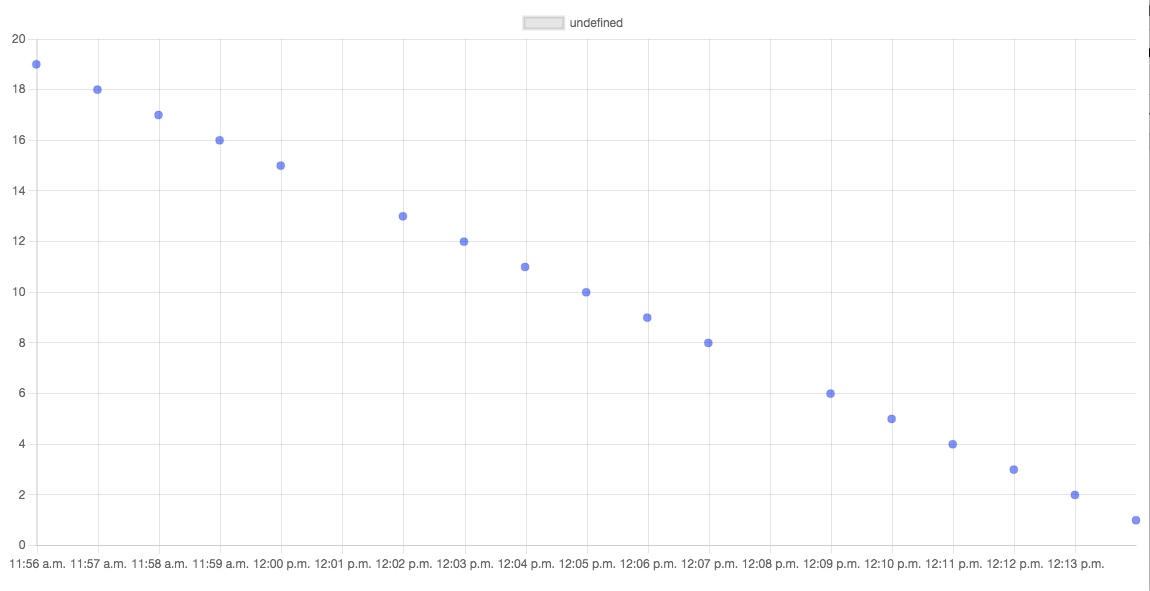In this graph, the thin vertical grid lines show minutes and the blue dots are our data points. Note that the blue dots are evenly spaced horizontally although they do not align with exact minutes. A reading that is taken every minute doesn’t require that it’s taken exactly at 0 seconds of that minute. We can see we’re missing a couple of points.

We can add these points when reading the data using `\$densify`. Although we will not initially have a value for them, we can at least create placeholder documents with the correct timestamp.

To do this, we read the data using a two stage aggregation pipeline as below, specifying the field we need to add, the magnitude of the time between readings, and whether we wish to apply it to some or all of the data points. We can also have separate scales based on data categories adding missing data points for each distinct airplane or sensor, for example. In our case, we will apply to all the data as we are reading just one metric in our simple example.

``1let densify = { \$densify : { field: "timestamp", 2                             range: { step: 1, unit: "minute", bounds: "full" }}}34let projection = {\$project: {_id:0, y: {\$ifNull:["\$reading",0]},x:"\$timestamp"}}56let tsdata = db.data.aggregate([densify,projection]).toArray()78graphTime(tsdata)``

This pipeline adds new documents with the required value of timestamp wherever one is missing. It doesn’t add any other fields to these documents, so they wouldn’t appear on our graph. The created documents look like this, with no reading or _id field.

``1{2  timestamp : ISODate("2022-03-23T17:55:32.485Z")3}``

To fix this, I have followed that up with a projection that sets the reading to 0 if it does not exist using `\$ifNull`. This is called zero filling and gives output like so.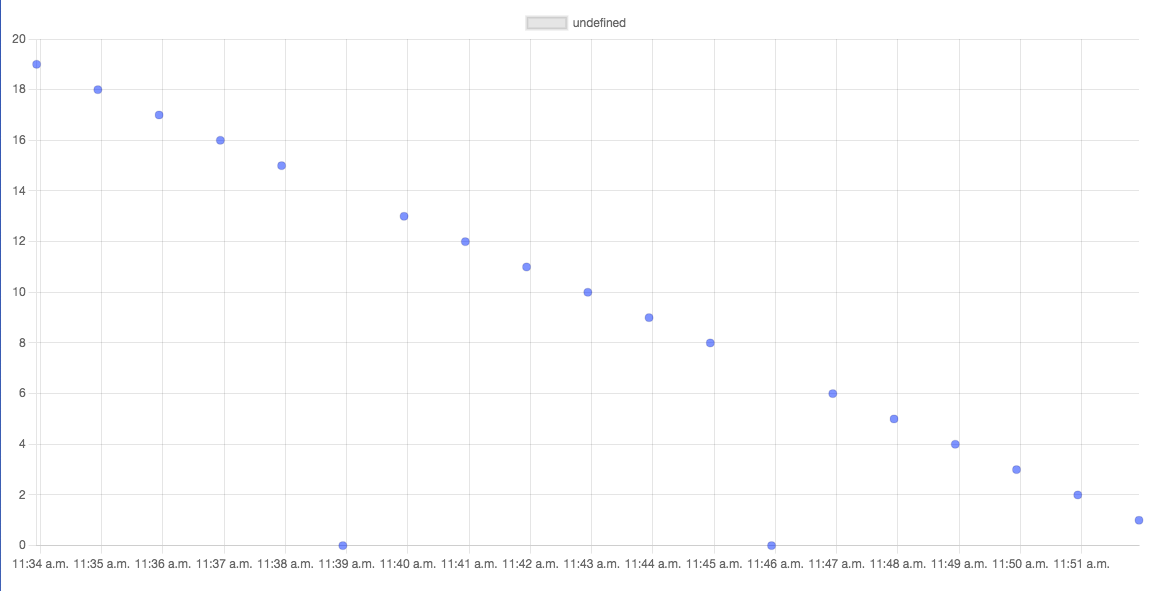To be useful, we almost certainly need to get a better estimate than zero for these missing readings—we can do this using `\$fill`.

## #Using \$fill to approximate missing readings

The aggregation stage `\$fill` was added in MongoDB 5.3 and can replace null or missing readings in documents by estimating them based on the non null values either side (ignoring nulls allows it to account for multiple missing values in a row). We still need to use `\$densify` to add the missing documents in the first place but once we have them, rather than add a zero reading using `\$set` or `\$project`, we can use `\$fill` to calculate more meaningful values.

To use `\$fill`, you need to be able to sort the data in a meaningful fashion, as missing readings will be derived from the readings that fall before and after them. In many cases, you will sort by time, although other interval data can be used.

We can compute missing values like so, specifying the field to order by, the field we want to add if it's missing, and the method—in this case, `locf`, which repeats the same value as the previous data point.

``1let densify = { \$densify : { field: "timestamp", 2                             range: { step: 1, unit: "minute", bounds : "full" }}}34let fill = { \$fill : { sortBy: { timestamp:1}, 5                       output: { reading : { method: "locf"}}}} 67let projection = {\$project: {_id:0,y:"\$reading" ,x:"\$timestamp"}}89let tsdata = db.data.aggregate([densify,fill,projection]).toArray()1011graphTime(tsdata)``

This creates a set of values like this.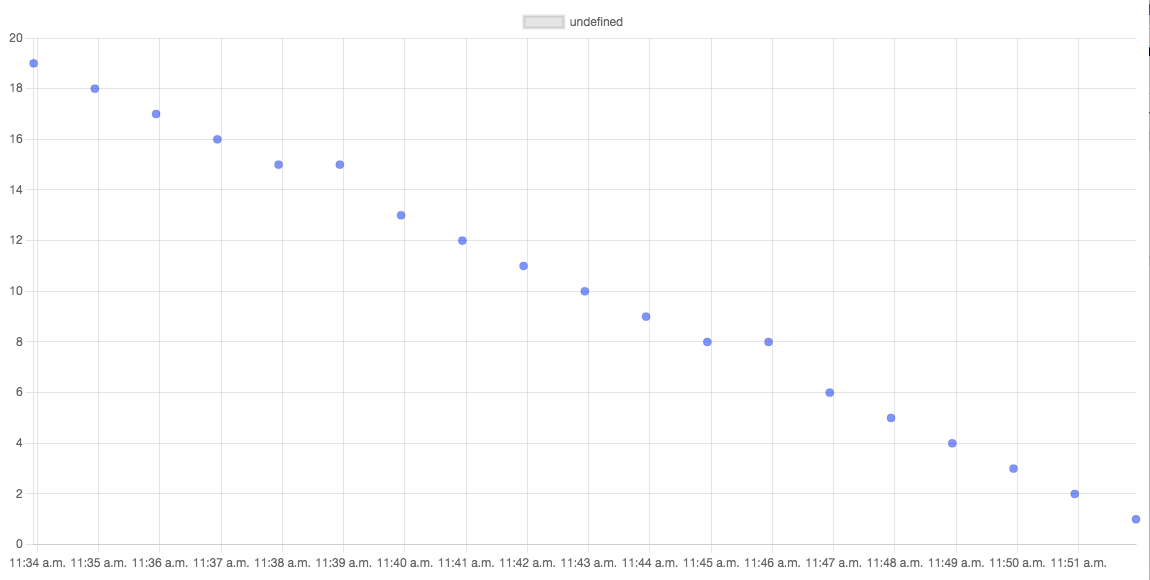In this case, though, those added points look wrong. Simply choosing to repeat the prior reading isn't ideal here. What we can do instead is apply a linear interpolation, drawing an imaginary line between the points before and after the gap and taking the point where our timestamp intersects that line. For this, we change `locf` to `linear` in our `\$fill`.

``1let densify = { \$densify : { field: "timestamp", 2                            range : { step: 1, unit: "minute", bounds : "full" }}}34let fill = { \$fill : { sortBy: { timestamp:1},5                       output: { reading : { method: "linear"}}}} 67let projection = {\$project: {_id:0,y:"\$reading" ,x:"\$timestamp"}}89let tsdata = db.data.aggregate([densify,fill,projection]).toArray()10graphTime(tsdata)``

Now we get the following graph, which, in this case, seems much more appropriate.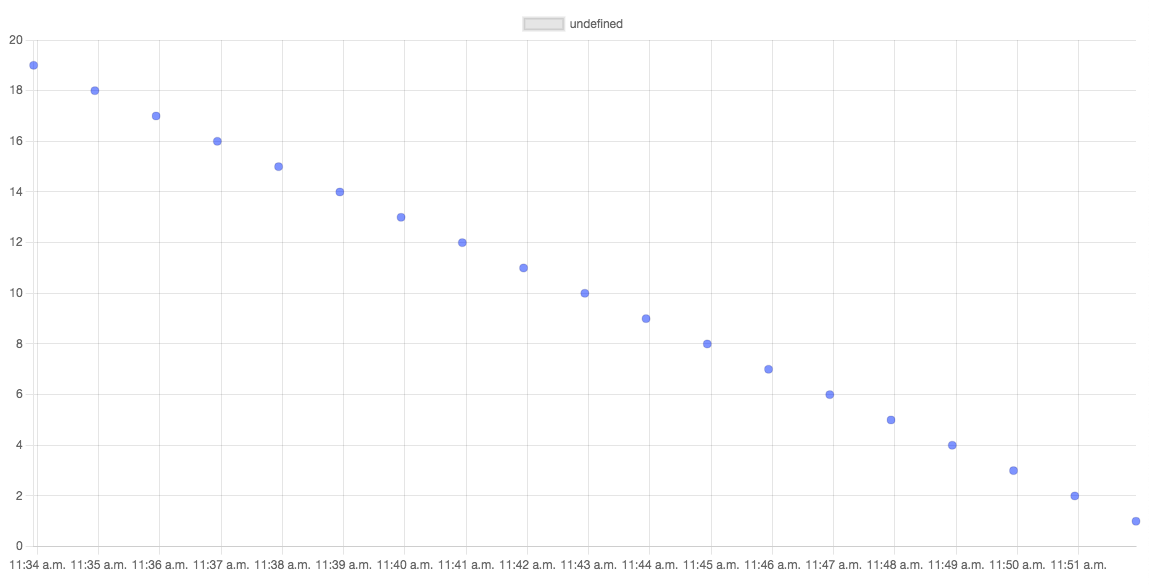We can see how to add missing values in regularly spaced data but how do we convert irregularly spaced data to regularly spaced, if that is what our analysis requires?

## #Converting uneven to evenly spaced data with \$densify and \$fill

Imagine we have a data set where we get a reading approximately once a minute, but unevenly spaced. Sometimes, the time between readings is 20 seconds, and sometimes it's 80 seconds. On average, it's once a minute, but the algorithm we want to apply to it needs evenly spaced data. This time, we will create aperiodic data like this in the mongosh shell spanning the previous 20 minutes, with some variation in the timing and a steadily decreasing reading.

``1db.db.getSiblingDB('tsdemo')23db.data.drop()45let timestamp =new  Date()6let start = timestamp;7for(let i=0;i<20;i++) {8   timestamp = new Date(timestamp.getTime() - Math.random()*60000 - 20000)9   let reading = (start-timestamp)/6000010   db.data.insertOne({reading,timestamp})11}``

When we plot this, we can see that the points are no longer evenly spaced. We require periodic data for our downstream analysis work, though, so how can we fix that? We cannot simply quantise the times in the existing readings. We may not even have one for each minute, and the values would be inaccurate for the time.

``1let tsdata = db.data.find({},{_id:0,y:"\$reading",x:"\$timestamp"}).toArray()23graphTime(tsdata)``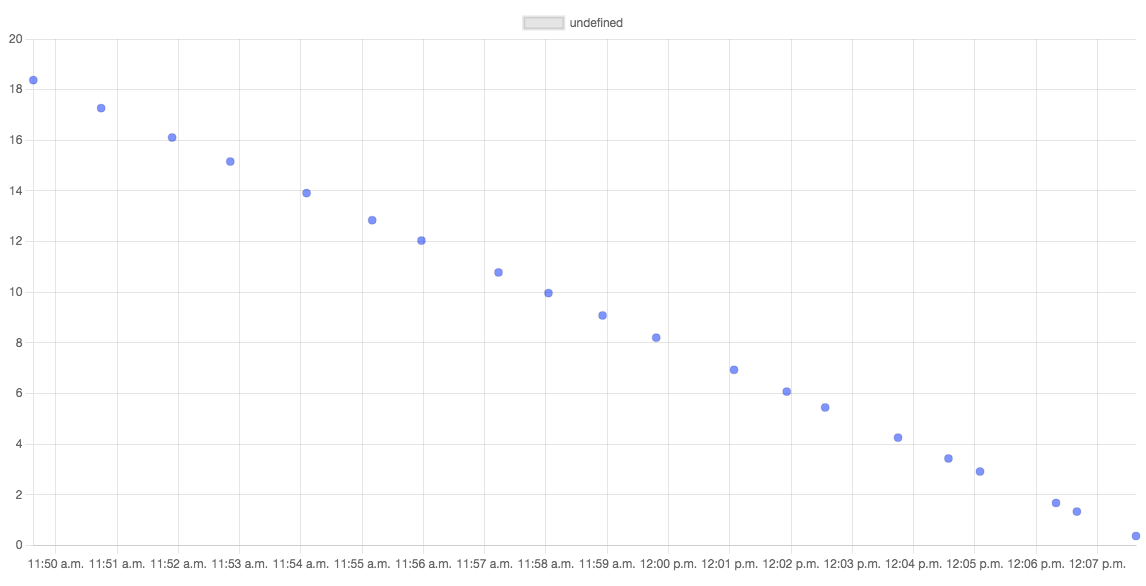We can solve this by using \$densify to add the points we require, \$fill to compute their values based on the nearest value from our original set, and then remove the original records from the set. We need to add an extra field to the originals before densification to identify them. We can do that with \$set. Note that this is all inside the aggregation pipeline. We aren’t editing records in the database, so there is no significant cost associated with this.

``1let flagOriginal = {\$set: {original:true}}23let densify = { \$densify: { field: "timestamp",4                             range: { step: 1, unit: "minute", bounds : "full" }}}567let fill = { \$fill : { sortBy: { timestamp:1},8                       output: { reading : { method: "linear"} }}} 910let projection = {\$project: {_id:0,y:"\$reading" ,x:"\$timestamp"}}1112let tsdata = db.data.aggregate([flagOriginal, densify,fill,projection]).toArray()13graphTime(tsdata)``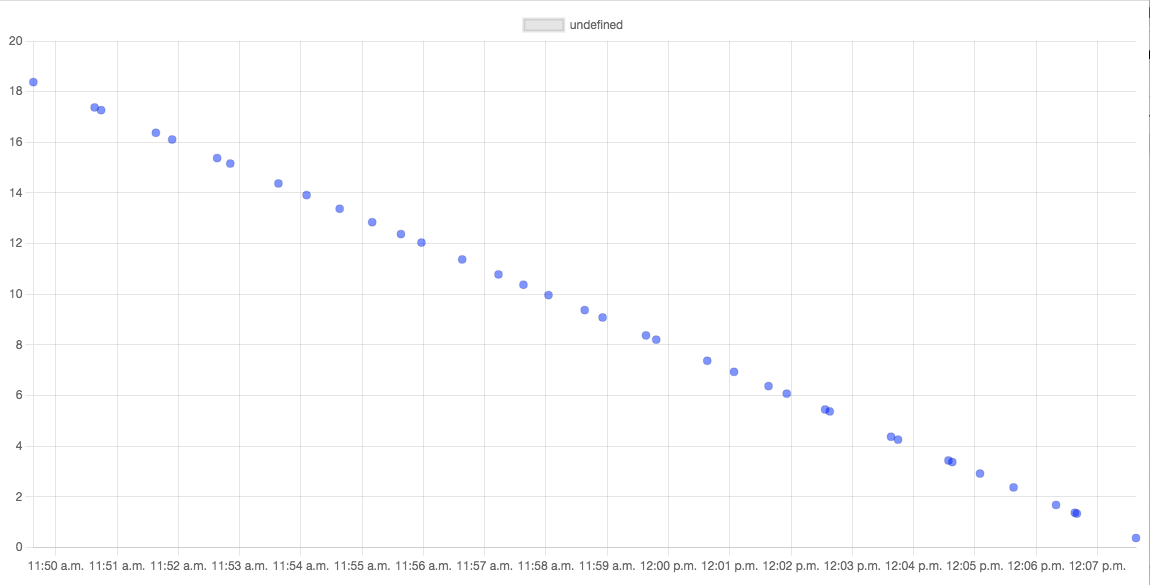We now have approximately double the number of data points, original and generated—but we can use \$match to remove those we flagged as existing pre densification.

``1let flagOriginal = {\$set : {original:true}}23let densify = { \$densify : { field: "timestamp",4                             range: { step: 1, unit: "minute", bounds : "full" }}}567let fill = { \$fill : { sortBy: { timestamp:1},8                       output: { reading : { method: "linear"} }}} 910let removeOriginal = { \$match : { original : {\$ne:true}}}1112let projection = {\$project: {_id:0,y:"\$reading" ,x:"\$timestamp"}}1314let tsdata = db.data.aggregate([flagOriginal, densify,fill,15                                removeOriginal, projection]).toArray()1617graphTime(tsdata)``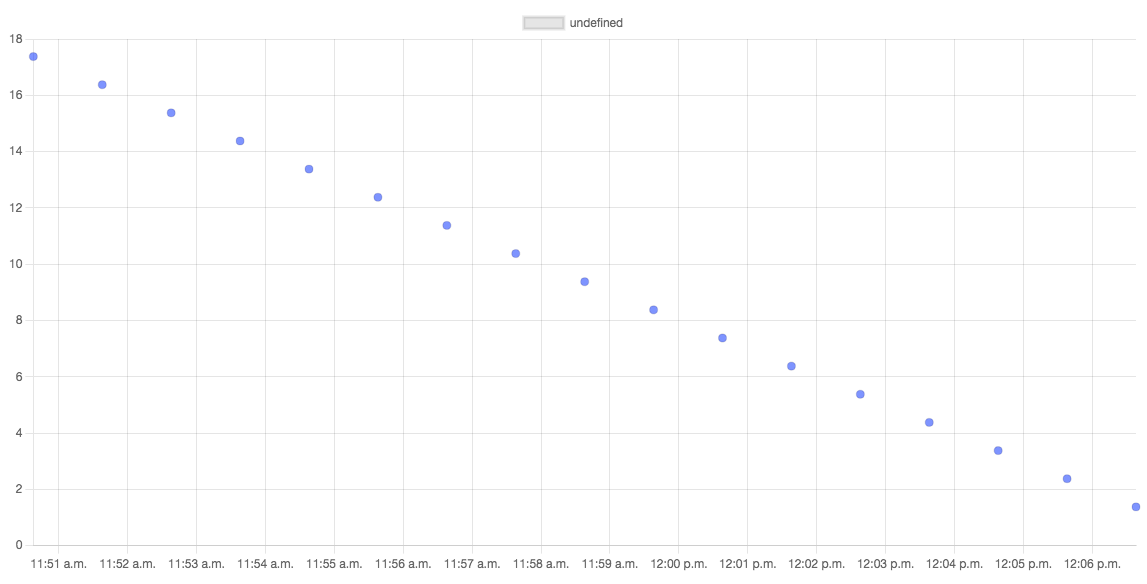Finally, we have evenly spaced data with values calculated based on the data points we did have. We would have filled in any missing values or large gaps in the process.

## #Conclusions

The new stages `\$densify` and `\$fill` may not initially seem very exciting but they are key tools in working with time series data. Without `\$densify`, there is no way to meaningfully identify and add missing records in a time series. The \$fill stage greatly simplifies the process of computing missing values compared to using `\$setWindowFields` and writing an expression to determine the value using the \$linear and \$locf expressions or by computing a moving average.

This then opens up the possibility of using a wide range of time series analysis algorithms in Python, R, Spark, and other analytic environments.